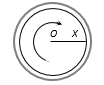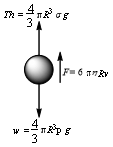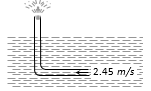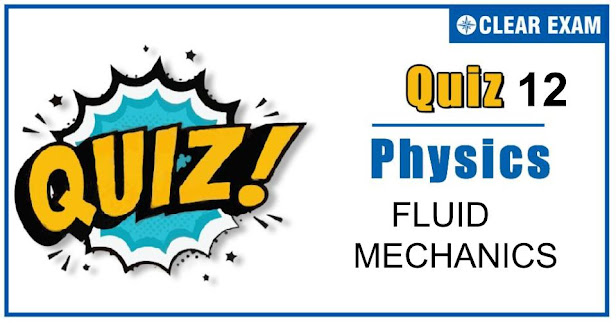## [LATEST]\$type=sticky\$show=home\$rm=0\$va=0\$count=4\$va=0

As per analysis for previous years, it has been observed that students preparing for NEET find Physics out of all the sections to be complex to handle and the majority of them are not able to comprehend the reason behind it. This problem arises especially because these aspirants appearing for the examination are more inclined to have a keen interest in Biology due to their medical background. Furthermore, sections such as Physics are dominantly based on theories, laws, numerical in comparison to a section of Biology which is more of fact-based, life sciences, and includes substantial explanations. By using the table given below, you easily and directly access to the topics and respective links of MCQs. Moreover, to make learning smooth and efficient, all the questions come with their supportive solutions to make utilization of time even more productive. Students will be covered for all their studies as the topics are available from basics to even the most advanced. .

Q1. The diagram shows a cup of tea seen from above. The tea has been stirred and is now rotating without turbulence. A graph showing the speed v with which the liquid is crossing points at a distance X from O along a radius XO would look likeSolution
When we move from centre to circumference, the velocity of liquid goes on decreasing and finally becomes zero

Q2.A sphere of mass M and radius R is dropped in a liquid, then terminal velocity of sphere is proportional to
•  R
•  1/R
•  R2
•  1/R2
Solution
If a sphere of mass M and radius R is dropped in a liquid, its weight Mg(=4/3 π R3 ρg) acts vertically downwards. While upthrust 4/3 π R^3 σg and viscous force 6πɳRv acts vertically upwards. Initially the body will be accelerated down. At a certain instant when viscous force F will balance the net downward force, acceleration will become zero and the body will fall with constant velocity.∴ 6πɳ RvT=4/3 π R3 (ρ-σ)g ie,vr=2/9 R2 ((ρ-σ)/ɳ)g or vT∝R2

Q3.  Streamline flow is more likely for liquid with
•  high density and low viscosity
•  low density and high viscosity
•  high density and high viscosity
•  low density and low viscosity
Solution
Streamline flow is more likely for non-viscous and incompressible liquid. So low density and low viscosity is the correct answer.

Q4. Water stands at level A in the arrangement shown in the figure. What will happen if a jet of air is gently blown into the horizontal tube in the direction shown in the figure?
•  Water will rise above A in the capillary tube
•  Water will fall below A in the capillary tube
•  There will be no effect on the level of water in the capillary tube
•  Air will emerge from end B in the form of bubbles
Solution
When air is blown in the horizontal tube, thepressure of air decreases in the tube. Due to which the water will rise above the tube A

Q5. Work done forming a liquid drop of radius R is W1 and that of radius 3R is W2. The ratio of work done is
•  1:3
•  1:2
•  1:4
•  1:9
Solution
W=T×4πR2 ⟹ W1/W1 =(T×4πR2)/(T×4π(3R)2 ) =(T×4πR2)/(T×36πR2 )=1/9 ∴ W1:W2=1:9

Q6.  Two bodies are in equilibrium when suspended in water from the arms of a balance. The mass of one body is 36 g and its density 9 g/cm^3. If the mass of the other is 48 g, its density in g/cm3 is
•  4/3
•  3/2
• 3
•  5
Solution
Apparent weight =V(ρ-σ)g=m/ρ (ρ-σ)g Where m= mass of the body ρ= density of the body σ= density of water If two bodies are in equilibrium then their apparent weight must be equal ∴m111-σ)=m222-σ)⇒36/9 (9-1)=48/ρ22-1) By solving we get ρ2=3

Q7. The working of an atomizer depends upon
•  Bernoulli’s theorem
•  Boyle’s law
•  Archimedes principle
•  Newton’s law of motion
Solution
Bernoulli’s theorem

Q8.  An L-shaped tube with a small orifice is held in a water stream as shown in fig. The upper end of the tube is 10.6 cm above the surface of water. What will be the height of the jet of water coming from the orifice? Velocity of water stream is 2.45 m/s•  Zero
•  20.0cm
•  10.6cm
•  40cm
Solution
According to Bernoulli’s theorem, h=v2/2g ⇒h=(2.45)2/(2×10)=0.30m=30.0cm ∴ Height of jet coming from orifice =30.0-10.6=19.4cm≅20cm

Q9. The surface energy of a liquid drop is u. It is sprayed into 1000 equal droplets. Then its surface energy becomes
•  u
•  10u
•  100u
•  1000u
Solution
Given : u=S×4 p R2; when droplet is splitted into 1000 droplets each of radius r, then 4/3 πR3=1000×4/3 πr3or r=R/10 ∴ Surface energy of all droplets =S×1000×4πr2=S×1000×4π(R/10)2 =10 (S 4π/R2)=10u

Q10. In making an alloy, a substance of specific gravity s1 and mass m1 is mixed with another substance of specific gravity s2 and mass m2 : then the specific gravity of the alloy is
•  ((m1+m2)/(s1+s2 ))
•  ((s1 s2)/(m1+m2 ))
•  (m1+m2)/(m1/s1 +m2/s2 )
• (m1/s1 +m2/s2 )/(m1+m2 )
Solution
Specific gravity of alloy =(Density of alloy)/(Density of water) =(Mass of alloy)/(Volume of alloy×density of water) =(m1+m2)/((m11 +m22)×ρw )=(m1+m2)/(m1/(ρ1w)+m2/(ρ2w ))=(m1+m2)/(m1/s1 +m2/s2 ) [As specific gravity of substance=(density of substance)/(density of water)]## Want to know more

Please fill in the details below:

Name